### 2.1.1 Fermi Theory of the Beta Decay

In the generic decay n->pea neutron couples to a vector field and hence becomes a proton while the incoming neutrino turns into an electron. In this picture at a single-point in space time the neutron wavefunction is transformed into that of the proton as the neutrino wavefunction transforms into that of the electron, since the anti-neutrino can be regarded as a neutrino that travels backward in time. The strength of the coupling in the single-point has to be obtained experimentally.

Fermi's idea was to compare the four-fermion point interaction as an interaction of two currents in analogy to the electromagnetic interaction. For proton-electron scattering, for instance, the matrix-element can be written as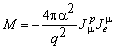.

J represents the current densities for proton and electron, respectively, and q the momentum transfer. Here the proton couples to the electron with the strength a which is known as the fine structure parameter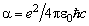. Fermi consequently introduced the weak current that decomposes into a hadronic and a leptonic current. Using the picture of second quantization (field quantization) the transition operator can be pictured as annihilator of the particle of the right hand side and creator of the particle on the left hand side. In the theory of four-fermion point interaction two particles are transformed simultaneously and therefore one writes the matrix element [Fey58]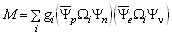.
The operators W i are denoted accordingly to their behaviour under parity transformations (see below) accompanied with their coupling constants gi. [Psi] represent the wave functions of the fermions participating in the b -decay, since they are solutions of the Dirac equation for free particles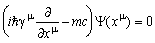.

In order to describe observables (which must be real expressions) in the Dirac theory, bilinear forms like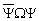with W being a 4x4 hermitean matrix, have to be generated. This results in 16 linear independent basic matrices which are be classified as follows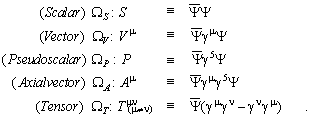with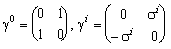,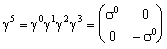(i=1,2,3)
where s i are the Pauli spin matrices and 1 the unit matrix.

This set of matrices guarantees that solutions of the Dirac equation also will fulfil Lorentz-invariance. The parity operator can be introduced by building the chiral projection operators: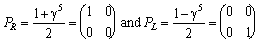one sees that with the help of these operators one can project the chirality state of the spinor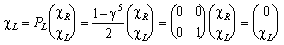(and analogous for the right-handed component).

The chiral symmetry was introduced by Feynman and Gell-Mann to account for parity violation, which was not known to Fermi. It can be shown that only V- and A-coupling correctly describe the maximal parity violation, since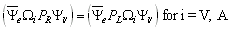.

Using this, the matrix element becomes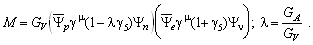In this form one sees that axial coupling is affected by the strong interaction, while the pure leptonic process couples with the strength Gv. This also is an important claim of the CVC-Hypothesis, namely that the form factors of leptons are unity and therefore are not renormalized by strong interaction. The CVC hypothesis is a direct consequence of the analogy to the electromagnetic interaction, as there is conservation of charge. As a result the coupling of the lepton current to the hadron current must be of equal strength not regarding whether there are nucleons or pions. So the Pion Beta decay rate is calculable like above.

#### 2.1.1.1 Beta Decay, Parity Violation and V-A Theory

The evidence for parity violation in b -decay has been established by two epochal experiments shortly after Lee and Yang formulated its possible occurrence. Wu et al. [Wu57] discovered the polarisation asymmetry of electrons in the b -decay of polarised 60Co; a year later Goldhaber et al. [Gol58] found the complete polarisation of neutrinos by measuring the photon spin direction determined by the deexcitation of a 152Eu* nucleus after K-capture. In order to account for the chiral symmetry breaking of the weak interaction only left-handed fermions participate and the mediating particles must be vectors of spin 1 and left-handed, as well. As a consequence the transition operators must be of the (V-A) kind, since only vector- and axial vector- coupling correctly describes maximal parity violation.

A textbook example to verify (V-A) theory is the calculation of pion-decay into leptons, since parity violation in pion and muon decays was observed along with the nuclear b -decay measurements mentioned above. Following the four fermion interaction picture with replacing the pion by a hadronic current of an up-quark changing into a down quark in the single-point, which demands universality of the weak interaction. It claims an universal coupling constant for all lepton decay and scattering processes (the equivalence of the coupling strength for weak interacting hadronic particles is expressed in the CVC hypothesis). In this example of a pion decay into leptons the conservation of angular momentum then determines the spin direction of the charged leptons, which have to be opposite to the (anti-)neutrino since pions do not carry spin. Finally, regarding conservation of momentum, the charged leptons have the same chirality as the neutrinos. The helicity of the neutrino must be -1. Hence, neutrino helicity determines the chirality of the charged lepton. Due to the fact that the probability to find a massive particle with unfavourable helicity goes with 1- b , the rate of the decay p +->e+ n e should be lower than for p +->µ+ n µ. With the approximation me2/m p 2 <<1 the ratio of decay rates turns out to be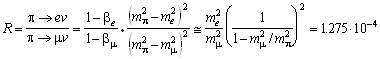,
where the first term describes the ratio of probabilities to generate a right-circular electron or muon, the second term represents the phase space difference. From the masses alone the p +->e+ n e decay would have been favoured by a factor of 5.5 (assuming scalar interaction)[Per87]. The experimental value is (1.267±0.023)*10-4 [PDG98] and thus confirms the validity of (V-A) interaction.

#### 2.1.1.2 The Beta Decay of Nuclei

The transition-rate of the process n->peis calculable by first order time dependent perturbation theory also known as Fermi's `golden rule', resulting in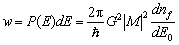.
Here w is the probability density for an electron to be emitted in the energy interval E+dE. The expression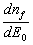represents the density of final state, M the transition matrix and G a combination of coupling constants. After a short calculation (see [Kal72] for example) and integration over the electron energies one obtains for the decay width: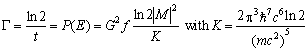.

The function f comprises the kinematic terms of the integrated state density and the electromagnetic interaction of the electrons and t represents the half-life. The connection of [florin]t-values, which are used to classify the b -decays, to the coupling constant one obtains after calculating the matrix element. Here the simplest case is a transition without a flip of the nucleon spin (I) and thus with anti-parallel electron and neutrino spins. An example of this pure Fermi transition is the inverse b -decay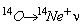. In order to calculate the matrix element one forms an isospin T=1 triplet of isotopes with the atomic number A=14. The isospin positions Tz are calculated assuming a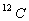core with additional nucleons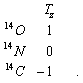Due to the spin pairing of the nucleons this triplet does not carry a net spin. Because of a transition without spin change (0->0) the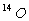decay is an example of a pure Fermi decay. Hence, the calculation of the matrix element M simplifies to sum over isospin T with Tz=1 in the initial state and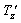=0 in the final. One obtains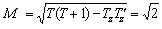and finally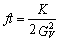,

since terms other than of vector type do not contribute Nuclear b -decays are classified according to their log([florin]t)-values:

 Type of transition D I parity log([florin]t) value super-allowed 0 + ~ 3.5 allowed 0,1, no 0->0 + ~ 5.7 (multiple) forbidden 0,1 or >1 - > 7.5
Table 2-1 Classification of b -decays [Mey84]. D I means the change of nuclear spin.

Before one can extract the exact coupling constant from the [florin]t-value, corrections have to be taken into account (see [Wil94,97] for a comprehensive discussion. Those are

* radiative corrections d r (from QED and QCD) of 3-4%

* nuclear correction d c of 0.2-1%

* screening effects due to Coulomb interaction with the core

Thus one writes f't = [florin]t (1+ d r) (1- d c). The rate of the pure Fermi nuclear b -decay is directly related to the vector coupling constant assuming the CVC hypothesis that claims the coupling to the hadronic current independent from the participating particles. Pions and nucleons therefore can be treated equally within this theory. Furthermore the standard model assumes the existence of an universal coupling constant for all weak processes. Hence, measuring the decay rate of a b -decay, GV can be obtained or, taking Gµ from the muon decay, universality of the weak interaction can be tested.

#### 2.1.1.3 Beta Decay of the Pion

Since pions are forming a spin 0 triplet with isospin T=1, a transition between two pions is analogous to the superallowed nuclear Fermi b -decay and therefore the pion beta decay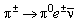can be described in the same way. Opposed to the nuclear b -decay neither inner nor outer nuclear corrections have to be taken into account; only radiative corrections (calculable with an accuracy of 0.2% [Sir92]) have to be considered. Thus the pion beta decay rate can be calculated with higher precision. A simple estimation of the pion beta decay rate can be done using the Sargent rule that asserts the dependence of the decay probability over time to the fifth power of the energy[Per86]. This gives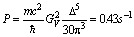,
since the pion mass difference D is the source of the transition energy (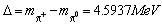[Cza93]). With an exact calculation including radiative corrections one gets after Källén [Kal72]: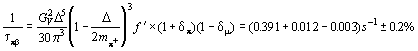.

(A more recent calculation by Sirlin [Sir92] gives (0.3996±0.0006) s-1).

Here the function f' is representing the integrated state density including electroweak corrections, d p the radiative corrections for the p of 0.012, and d µ.represents the radiative correction for the µ+->e+ n edecay. With the pion lifetime of 26.03 ns this is equivalent to a branching ratio (BR) of 1.031*10-8 or a ratio of 0.838*10-4 compared to our calibration decay p +->e+ n e .

 Here the Weyl representation is used.